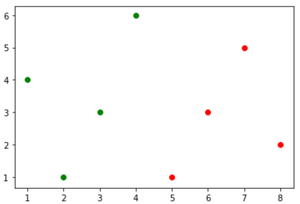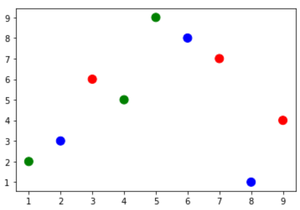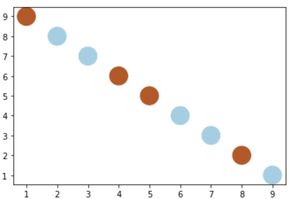# How to create a Scatter Plot with several colors in Matplotlib?

• Last Updated : 07 Feb, 2022

Matplotlib is a plotting library for creating static, animated, and interactive visualizations in Python. Matplotlib can be used in Python scripts, the Python and IPython shell, web application servers, and various graphical user interface toolkits like Tkinter, awxPython, etc.

In-order to create a scatter plot with several colors in matplotlib, we can use the various methods:

Method #1: Using the parameter marker color i.e. c

The possible values for marker color are:

• A single color format string.
• A 2-D array in which the rows are RGB or RGBA.

Example:

Using the c parameter to depict scatter plot with different colors.

## Python3

 `# import required module``import` `matplotlib.pyplot as plt` `# first data point``x ``=` `[``1``, ``2``, ``3``, ``4``]``y ``=` `[``4``, ``1``, ``3``, ``6``]` `# depict first scatted plot``plt.scatter(x, y, c``=``'green'``)` `# second data point``x ``=` `[``5``, ``6``, ``7``, ``8``]``y ``=` `[``1``, ``3``, ``5``, ``2``]` `# depict second scatted plot``plt.scatter(x, y, c``=``'red'``)` `# depict illustration``plt.show()`

Output:Method #2: Using the colormap

Colormap instances are used to convert data values (floats) from the interval [0, 1] to the RGBA color.

Example 1:

Using the colormap to depict scatter plot with RGB colors.

## Python3

 `# import required modules``import` `matplotlib.pyplot as plt``import` `numpy` `# assign data points``a ``=` `numpy.array([[``9``, ``1``, ``2``, ``7``, ``5``, ``8``, ``3``, ``4``, ``6``],``                 ``[``4``, ``2``, ``3``, ``7``, ``9``, ``1``, ``6``, ``5``, ``8``]])` `# assign categories``categories ``=` `numpy.array([``0``, ``1``, ``2``, ``0``, ``1``, ``2``, ``0``, ``1``, ``2``])` `# use colormap``colormap ``=` `numpy.array([``'r'``, ``'g'``, ``'b'``])` `# depict illustration``plt.scatter(a[``0``], a[``1``], s``=``100``, c``=``colormap[categories])``plt.show()`

Output:Example 2:

Here, we manually assign the colormap using color codes.

## Python3

 `# import required modules``import` `matplotlib.pyplot as plt``import` `numpy` `# assign data points``a ``=` `numpy.array([[``1``, ``2``, ``3``, ``4``, ``5``, ``6``, ``7``, ``8``, ``9``],``                 ``[``9``, ``8``, ``7``, ``6``, ``5``, ``4``, ``3``, ``2``, ``1``]])` `# assign categories``categories ``=` `numpy.array([``0``, ``1``, ``1``, ``0``, ``0``, ``1``, ``1``, ``0``, ``1``])` `# assign colors using color codes``color1 ``=` `(``0.69411766529083252``, ``0.3490196168422699``,``          ``0.15686275064945221``, ``1.0``)``color2 ``=` `(``0.65098041296005249``, ``0.80784314870834351``,``          ``0.89019608497619629``, ``1.0``)` `# assign colormap``colormap ``=` `numpy.array([color1, color2])` `# depict illustration``plt.scatter(a[``0``], a[``1``], s``=``500``, c``=``colormap[categories])``plt.show()`

Output:My Personal Notes arrow_drop_up